### Home > CALC > Chapter 5 > Lesson 5.2.5 > Problem5-105

5-105.
1. You can use a tangent line to estimate the value of a function at points near the point of tangency. Homework Help ✎

1. Sketch a graph of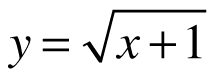and its tangent line at x = 0. Then find the equation of this tangent line.

2. Use the tangent to approximate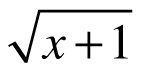for x = 0.1 and x = −0.1.

3. Use your calculator to evaluate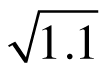and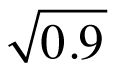. Find the percentage error for your estimate in each case. How accurate was your approximation?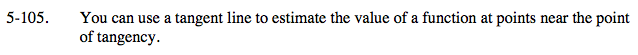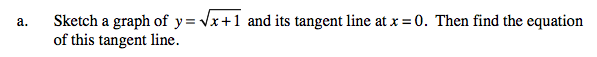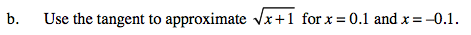One purpose of tangent lines:

Since evaluating square roots is more challenging than evaluating straight lines, and since the straight line (tangent line) is very close to the square root near its point of tangency, we will evaluate the tangent line at x = 0.1 and x = −0.1 in order to approximate the values of the square root graph.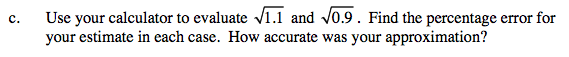Use the eTool below to visualize the problem.
Click the link at right for the full version of the eTool: Calc 5-105 HW eTool